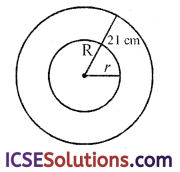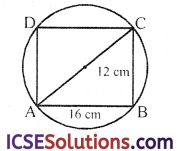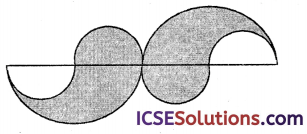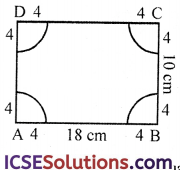Students often turn to S Chand Class 9 Maths Solutions ICSE Chapter 17 Circle: Circumference and Area Chapter Test to clarify doubts and improve problem-solving skills.

## S Chand Class 9 ICSE Maths Solutions Chapter 17 Circle: Circumference and Area Chapter Test

Question 1.
A cycle wheel makes 1000 revolutions in moving 440 m. What is the diameter of the wheel?
(a) 7 cm
(b) 14 cm
(c) 28 cm
(d) 21 cm
Solution:
Number of revolutions = 1000
Distance travelled = 440 m
∴ Perimeter of the wheel = $$\frac{440 \times 100}{1000}$$ = 44 cm
and diameter = $$\frac{\text { Perimeter }}{\pi}$$
= $$\frac{44 \times 7}{22}$$ = 14 cmQuestion 2.
The area of a semi-circular fiels is 308 sq m; then taking π = $$\frac { 22 }{ 7 }$$, the length of the railing to surround it has to be
(a) 88 cm
(b) 80 cm
(c) 44 cm
(d) 72 cm
Solution:
Area of a semi-circular field = 308 sq mPerimeter = $$\frac { 1 }{ 2 }$$ × 2πr + 2r = πr + 2r = $$\frac { 22 }{ 7 }$$ × 14 + 2 × 14
= 44 + 28 = 72 cm

Question 3.
A wire of length of 36 cm is bent in the form of a semi-circle. What is the radius of the semi-circle?
(a) 9 cm
(b) 8 cm
(c) 7 cm
(d) 6 cm
Solution:
Length of wire = 36 cm
∴ Circumference of semi-circle = 36 cm
∴ πr + 2r = 36
⇒ $$\frac { 22 }{ 7 }$$r + 2r = 36 ⇒ $$\frac { 36 }{ 7 }$$r = 36
⇒ r = $$\frac{36 \times 7}{36}$$ = 7 cm

Question 4.
A wire is in the form of a circle of radius 42 cm. If it is bent into a square, then what is the side of the square?
(a) 66 cm
(b) 42 cm
(c) 36 cm
(d) 33 cm
Solution:
Radius of a circular wire = 42 cm
∴ Its perimeter = 2 πr
=2 × $$\frac { 22 }{ 7 }$$ × 42 cm = 264 cm
∴ Perimeter of square = 264 cm
Side = $$\frac{\text { Perimeter }}{4}$$ = $$\frac { 264 }{ 4 }$$ = 66 cm

Question 5.
A metal wire when bent in the form of a square encloses an area 484 cm2. If the same wire is bent in the form of a circle, then its area is $$\left(\text { Take } \pi=\frac{22}{7}\right)$$
(a) 616 cm2
(b) 5040 cm2
(c) 1232 cm2
(d) 2464 cm2
Solution:
Area of square metal wire = 484 cm2
∴ Side = $$\sqrt{\text { Area }}$$ = $$\sqrt{484}$$ cm = 22 cm
∴ Perimeter = 4 × Side = 4 × 22 = 88 cm
∴ Perimeter of circle = 88 cm
∴ Radius of circle = $$\frac{\text { Perimeter }}{2 \pi}$$
= $$\frac{88 \times 7}{2 \times 22}$$ = 14 cm
and area = πr2 = $$\frac { 22 }{ 7 }$$ × 14 × 14 cm2
= 616 cm2

Question 6.
In the figure, the area enclosed between the two co-centric circles is 770 cm2. If the radius of the outer circle is 21 cm, the radius of the inner circle is(a) 14 cm
(b) 22 cm
(c) 12 cm
(d) 10.5 cm
Solution:
In the figure, enclosed area between the circles = 770 cm2
Radius of outer circle (R) = 21 cm
Let radius of inner circle (r) = r
Area of circles = π[R2 – r2]Question 7.
A circle circumscribes a rectangle with side 16 cm and 12 cm. What is the area of the circle?(a) 48 π sq cm
(b) 50 π sq cm
(c) 100 π sq cm
(d) 200 π sq cm
Solution:
A circle is circumscribed by a rectangle with sides 16 cm and 12 cm∴ Diagonal AC = $$\sqrt{\mathrm{AB}^2+\mathrm{BC}^2}$$
= $$\sqrt{16^2+12^2}$$ = $$\sqrt{256+144}$$ cm
= $$\sqrt{400}$$ = 20 cm
∴Diameter of circle = 20 cm
and radius = $$\frac { 20 }{ 2 }$$ = 10 cm
Area of circle = πr2 = π × 10 × 10 = 100π cm2Question 8.
A person rides a bicycle round a circular path of radius 50 m. The radius of the wheel of the bicycle is 50 cm. The cycle comes to the starting point for the first time in 1 hour. What is the number of revolutions of the wheel in 15 min?
(a) 20
(b) 25
(c) 30
(d) 35
Solution:
Radius of circular path (R) = 50 m and radius of wheel of a bicycle = 50 cm Distance travelled by wheel of a cycle = 1 h Now circumference of path = 2πR
= $$\frac{2 \times 22}{7}$$ × 50 m = $$\frac{2200}{7}$$m
and circumference of wheel
= $$\frac{2 \times 22}{7}$$ × 50 cm = $$\frac{2200}{7}$$cm
∴Number of revolutions = $$\frac{2200 \times 100 \times 7}{7 \times 2200}$$ = 100
Number of revolution in 15 minutes
= $$\frac{100 \times 15}{60}$$ = 25 (1 hr = 60 min.)

Question 9.
The figure consists of four small semi-circles of equal radii (each 42 cm) the perimeter of the shaded region is(a) 524 cm
(b) 264 cm
(c) 396 cm
(d) 504 cm
Solution:
The given figure consists of 4 small semicircles
Radii of each small semi-circle = 42 cm and radius of big semi-circles = 42 × 2 = 84 cm= 4 × πr + 2 × πR
= 4 × $$\frac{22}{7}$$ × 42 + 2 × $$\frac{22}{7}$$ × 84 cm
= 528 + 528 = 1056 cm

Question 10.
A rectangular cardboard is of dimensions 18 cm × 10 cm. From the four corners of the rectangle quarter circles of radius 4 cm are cut. What is the perimeter (approximate) of the remaining portion?
(a) 47.1 cm
(b) 49.1 cm
(c) 51.0 cm
(d) 53.0 cm
Solutiion:=4 × $$\frac{1}{2}$$ × πr
= 2 × $$\frac{22}{7}$$ × 4 = $$\frac{176}{7}$$ = 25.1 cm